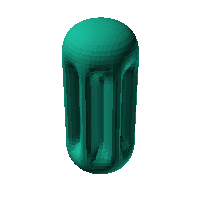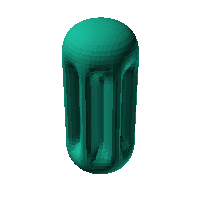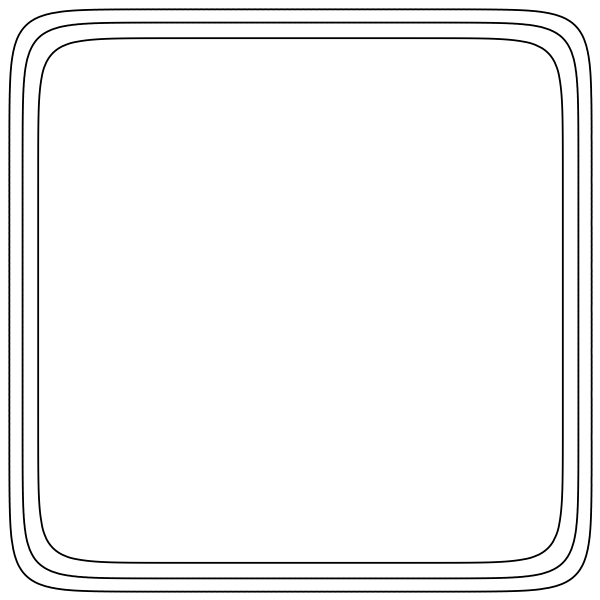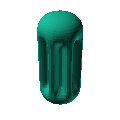Blob Farm# blob25The blob in all its glory:pleated pill shape.

 Click on the snapshot to download the blob's stl file.Octave Code:
```  # name of the blob
project = "blob25";

function w = f(x2,y2,z2,c,r,e)
x  = (x2-c(1))/r(1);
y  = (y2-c(2))/r(2);
z  = (z2-c(3))/r(3);
# function at origin must be <0, and >0 far enough away.  w=0 defines the surface
th = atan2(x,y);

w = -(1./(sqrt((x/40).^2+(y/40).^2+(z/90).^8) + 0.2*(1+cos(th*7)-0.115*cos(21*th))./(1+(z/52).^12)))+1;

endfunction;

step = 4;  # grid pitch in mm  start with 4mm to see the shape quickly.  Once you have it just right, change to 2mm for printing

c_outer = [0,0,0];
r_outer = [1,1,1];

source("../octave/func2stl_v01.m");  # do all the calculations
```
GNU Octave